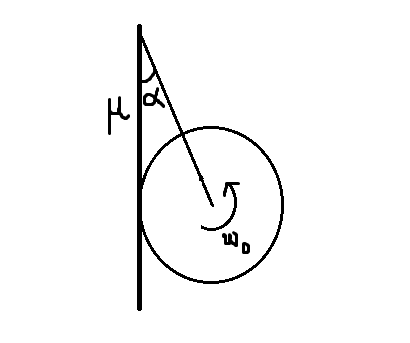# The Ring is retarded to 0 rad/sConsider a ring of mass $m$ and radius $R$ touching a rough wall of coefficient of friction $\mu$ as shown in figure. The ring has an initial angular velocity of $\omega_0$.

Find the time taken by the ring to come to a halt.

Details and Assumptions

• The ring doesn't translate on the wall.

• $\mu = \dfrac{1}{\sqrt{3}}$

• $m = 1 kg$

• $R = 1 m$

• $\alpha = \dfrac{\pi}{3}$

• $\omega_0 = 10 rad/s$

• $g = 10 m/s^2$

×